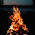## Sunday, December 06, 2015

### Arithmetic Progression - Nth Term, Sum, Middle Term, Corresponding Term, Increasing and Decreasing AP, Average Explained Video Lecture

Friends in this video I have started with the topic of Progressions and first I have covered the whole topic of Arithmetic Progression in full detail. The definition of Arithmetic Progression - When a particular pattern is displayed by numbers of a series due to addition or substraction of a constant in subsequent numbers then it is termed as an Arithmetic Progression. We normally suppose the first term of an Arithmetic Progression as a and the common difference as d. I have explained that Arithmetic Progression can be of two types - Increasing and Decreasing. I have explained the way of deriving the formula of Nth term of an Arithmetic Progression which comes out to be (a +(N-1)d). I have also explained how to prove the formula of sum of an Arithmetic Progression. Then I have taken up the concept of middle term in an Arithmetic Progression of odd and even number of terms. After that I have explained what is corresponding pair in an Arithmetic Progression and how the average of the whole Arithmetic Progression is calculated from it. Also finding out the average through the middle term of an Arithmetic Progression has also been explained. Then using that average to calculate the sum of the Arithmetic Progression has also been explained. Then a specific case of Arithmetic Progression has been explained by me in which I have told how to solve those questions which are of the type of Question Number 8 and 9 below. You have to watch this video repeatedly in order to command over these concepts and use them efficiently while solving questions. Your feedback, comments and doubt are welcome in the comment section below.
Question 1. The 7th and 21st term of an Arithmetic Progression are 6 and -22 respectively. Find the 26th term of the same Arithmetic Progression ?

Question 2. Find the 15th term of the sequence - 20,15,10..................

Question 3. The sum of 5 numbers in Arithmetic Progression is 30 and the sum of their squares is 220. What is the 3rd term of this Arithmetic Progression ?

Question 4. Find the second term of an Arithmetic Progression if the sum of its first five even terms is equal to 15 and the sum of the first three terms is equal to -3.

Question 5. Find the sum of all three digit integers that are divisible by 7.

Question 6. In an Arithmetic Progression with odd number of terms, the sum of all the terms is 84. The middle term is 5, more than the number of terms. Find the number of terms ?

Question 7. An Arithmetic Progression has 200 terms. The nth term from the beginning is 15 and the nth term from the end is 45. What is sum of this series ?

Question 8. The sum of the first 12 terms of an Arithmetic Progression is equal to the sum of the first 18 terms of the same Arithmetic Progression. What will be the sum of the first 30 terms of the same Arithmetic Progression ? (CAT 2004)

Question 9. If the sum of the first 16 terms of an Arithmetic Progression is equal to the sum of the first 18 terms. Find the sum of the first 34 terms of the same Arithmetic Progression ?

Question 10. If the sum of the first n terms of an Arithmetic Progression is 3n2+ 6n , then the nth term of the Arithmetic Progression would be denoted by

Question 11. The sum to 100 terms of a series in Arithmetic Progression is 5050 and the 10th term is 10. What are the values of the first term and the common difference respectively ?

Question 12. The 69th term of an Arithmetic Progression is 16 times the fourth term of an Arithmetic Progression. Find the 20th term of this Arithmetic Progression, if the 7th term is 22 ?

Question 13. Find the 35th term of an Arithmetic Progression whose first and last term are respectively 4 and 241 and the sum of all terms is 9800 ?

Question 14. Find the sum of first 19 terms of an Arithmetic Progression, if the sum of the first, the third, the eighth, the twelfth, the seventeenth and the nineteenth term is 555 ?

1.nice lecture ,help me a lot.thnks sir

2.3.4.